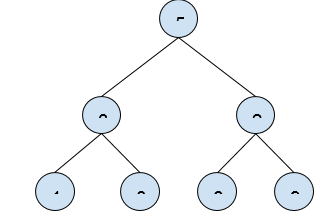# Find maximum (or minimum) in Binary Tree in C++

In this problem, we are given a binary tree. Our task is to Find maximum (or minimum) in Binary Tree.

Problem Description: We need to find the nodes of the binary tree that have maximum and minimum value in the binary tree.

Let’s take an example to understand the problem,

Input:Output: max = 9 , min = 1

## Solution Approach

We need to find the max node of the binary tree. We will do this by traversing the pointer until we reach the leaf node and then find the maximum node of the tree.

## Example

Live Demo

#include <iostream>
using namespace std;

class Node {
public:
int data;
Node *left, *right;

Node(int data) {
this->data = data;
this->left = NULL;
this->right = NULL;
}
};

int findMaxNode(Node* root) {

if (root == NULL)
return -100;

int maxVal = root->data;
int leftMaxVal = findMaxNode(root->left);
int rightMaxVal = findMaxNode(root->right);
if (leftMaxVal > maxVal)
maxVal = leftMaxVal;
if (rightMaxVal > maxVal)
maxVal = rightMaxVal;
return maxVal;
}

int main() {

Node* NewRoot = NULL;
Node* root = new Node(5);
root->left = new Node(3);
root->right = new Node(2);
root->left->left = new Node(1);
root->left->right = new Node(8);
root->right->left = new Node(6);
root->right->right = new Node(9);
cout<<"The Maximum element of Binary Tree is "<<findMaxNode(root) << endl;
return 0;
}

## Output

The Maximum element of Binary Tree is 9